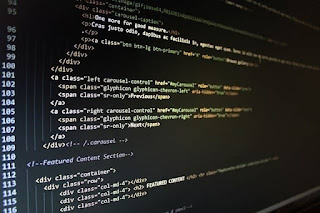In this tutorial we will discuss on function, their return type and arguments and types in C++ (CPP).

###Function:

→ A function is a self contained program segment or module that carries out a very specific and well defined task.

→ Following are different components of function:

1. Function Prototype

2. Function Call

3. Function Definition Function Prototype:

→ The function prototype informs the compiler about the return type of the function, function name and list of arguments.

→ The syntax for writing function prototype is: return_type

function_name(type1,type2,type3,… …,typen);
→ For example:
2. void copystring(char *,char *);
4. int findLength(char []);
6. float summer(float,int);
7. int sumnterms(int);
8. int calcLargest(int [],int);

→ If a function is defined before it is called, function prototype is not necessary.

→ Function prototype is important because it helps is programming documentation and type checking that might eliminate the probable run time errors.

→ We should explicitly include function prototype for every user defined function to increase type-checking capability of the compiler and use #include preprocessor directive for function prototypes of standard library functions.

### Function Call

→ The function call transfers the control from the calling function to called function with a list of arguments(passing arguments is optional).

→ The syntax for the function call is:

function_name(list_of_arguments);

→ The list of arguments mentioned in the function call are known as actual arguments.
→ When a function is called, the actual arguments are copied into corresponding formal arguments.
→ When value of actual arguments is passed, the calling mechanism is known as pass by value or call by value.
→ When the memory address of actual arguments is passed, the calling mechanism is known as pass by reference or call by reference.

### Function Definition

→ The function definition lists the code that fulfills the purpose for which the function is created.
→ The syntax for the function definition is:
return_type function_name(type1 arg1, type2 arg2, … …,typen argn)
{
statement(s);
return expression; //optional
}

→ The list of arguments declared in the header of function definition are known as formal arguments.
→ It is not necessary to re-declare the formal arguments in the function body.

### On the basis of return type and arguments, functions can be classified as:

i. Function with return type and argument
ii. Function with no return type and no argument
iii. Function with return type and no argument
iv. Function with no return type and argument

WAP in C++ to find the sum of two integers using function
with return type and arguments.

#include<iostream>
using namespace std;
int addition(int, int); //Function prototype int main() { int a,b,s;
cout<<"Enter two numbers:";
cin>>a>>b;
cout<<"The sum of the entered numbers is "<<s;
return 0; }
int addition(int p, int q) {     return p+q; }

Example 2:

WAP in C++ to find the sum of two integers using function with

no return type and no argument.
#include<iostream>
using namespace std;
int main()
{
{ int a,b;
cout<<"Enter two numbers";
cin>>a>>b;
cout<<"The sum of the entered numbers is "<<a+b;
}

Example 3:
WAP in C++ to find the sum of two integers using function
with return type and no arguments.
#include<iostream>
using namespace std;
int addition(); //Function prototype int main() { int s; s = addition(); //Function call cout<<"The sum of the entered numbers is "<<s;
return 0;
}
int addition() { int a,b; cout<<"Enter two numbers:";
cin>>a>>b;
return a+b;
}

Example 4:

WAP in C++ to find the sum of two integers using function
with no return type and arguments.
#include<iostream>
using namespace std;
int main()
{
int a,b;
cout<<"Enter two numbers:";
cin>>a>>b;
return 0; } void addition(int p,int q)
{
cout<<"The sum of the entered numbers is "<<p+q;
}

→ The ability of having more than one function in C++ with same function name but having differences in type/number of arguments is known as function overloading.

Example:
Example: WAP in C++ to calculate the area of circle and rectangle
#include<iostream>
#define PI 3.1414
using namespace std; float area(float);
float area(float,float);
cout<<"Enter length and breadth of rectangle"<<endl;
return 0; }
float area(float r) { return PI*r*r; }
float area(float l,float b) { return l*b; }

### Inline Functions

→ C++ provides an inline functions to reduce the function call overhead.
→ Inline function is a function that is expanded in line when it is called. When the inline function is called whole code of the inline function gets inserted or substituted at the point of inline function call.
→ This substitution is performed by the C++ compiler at compile time.
→ Inline function may increase efficiency if it is small.

→ The syntax for defining inline function is given below:

inline return_type function_name(type1arg1,type2 arg2,…,typen argn)
{
statement(s); return expression;
}

Example:
Example: WAP in C++ to illustrate the concept of inline function #include<iostream>
using namespace std; inline float calcSI(float p,float t,float r) {     return (p*t*r)/100; } int main() { float t=2.00,r=12;
cout<<"p"<<"\t"<<"t"<<"\t"<<"SI"<<endl;
cout<<"------------------"<<endl;
for(float p=100;p<=500;p=p+100) {     cout<<p<<"\t"<<t<<"\t"<<calcSI(p,t,r)<<endl; }
return 0; }

This is the end of function, their return type and arguments and types in C++ (CPP).